Examples

Chapter 2 Class 8 Linear Equations in One Variable
Serial order wise

To do this question, first check Two digit number and its reverse .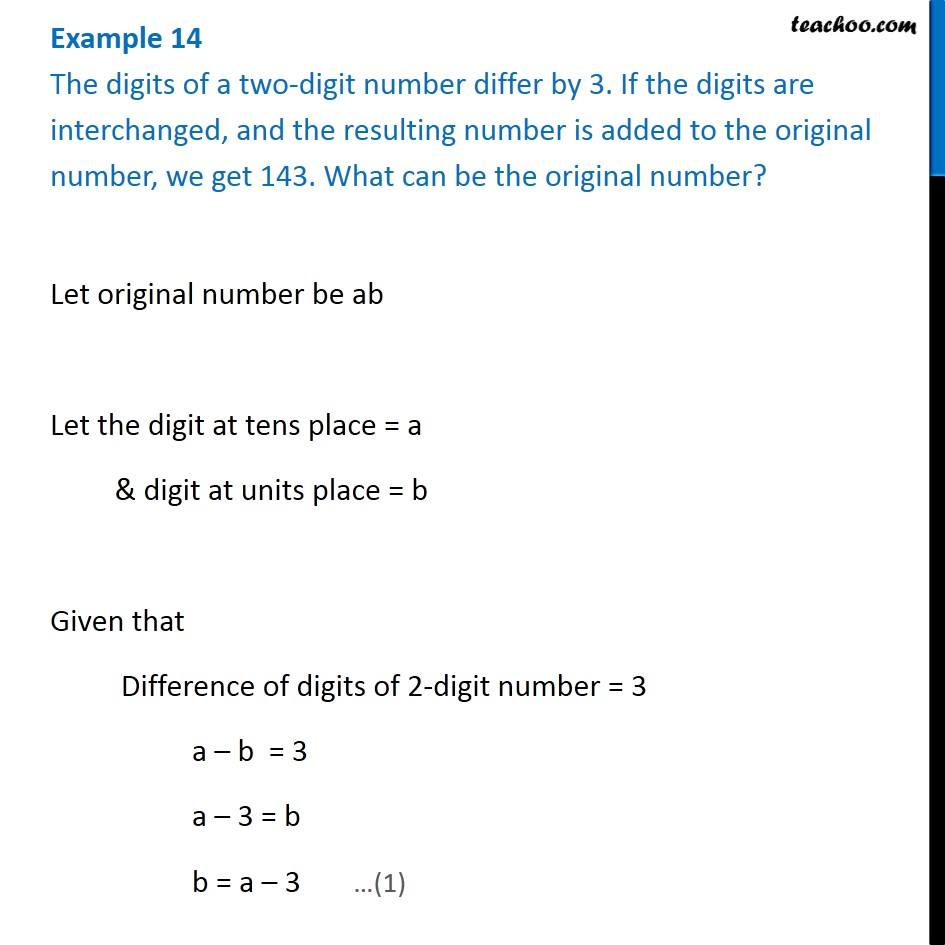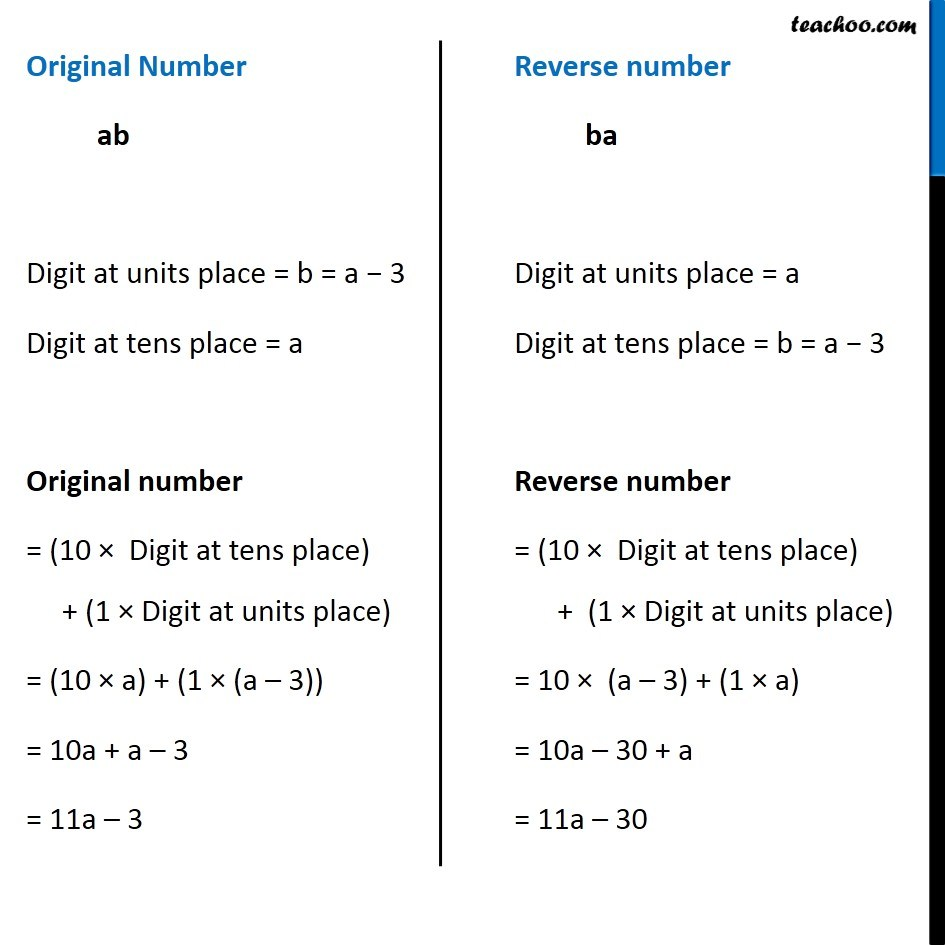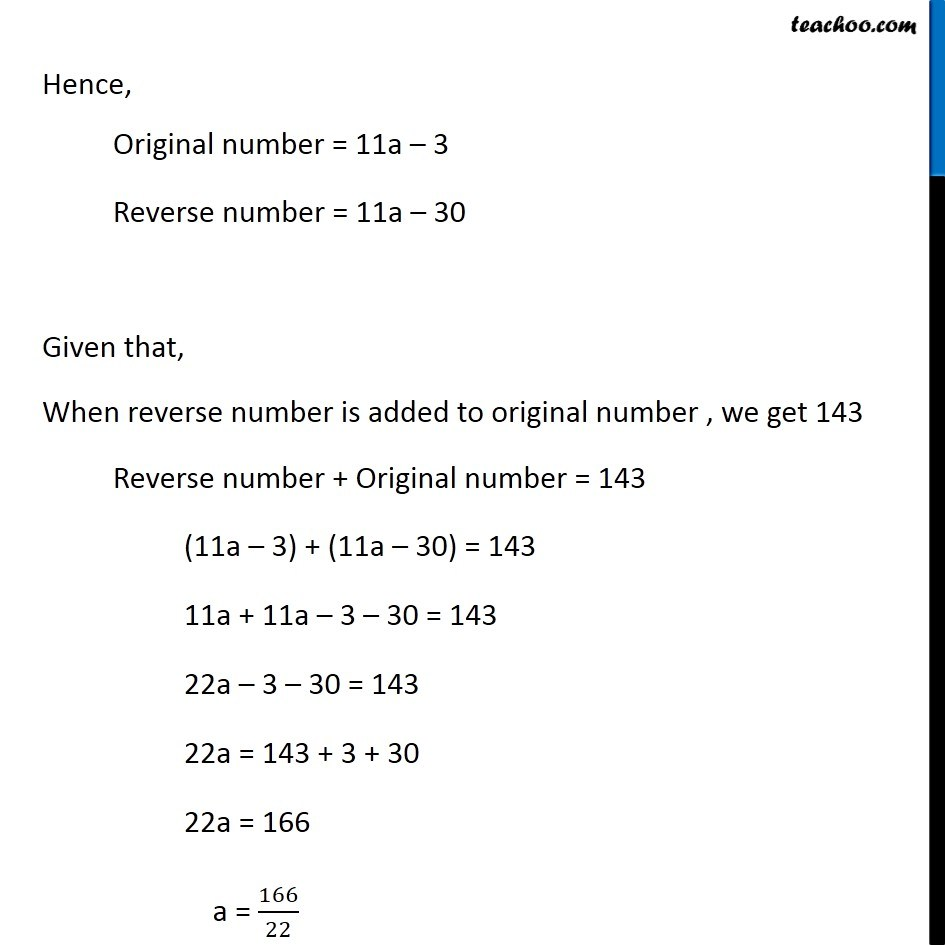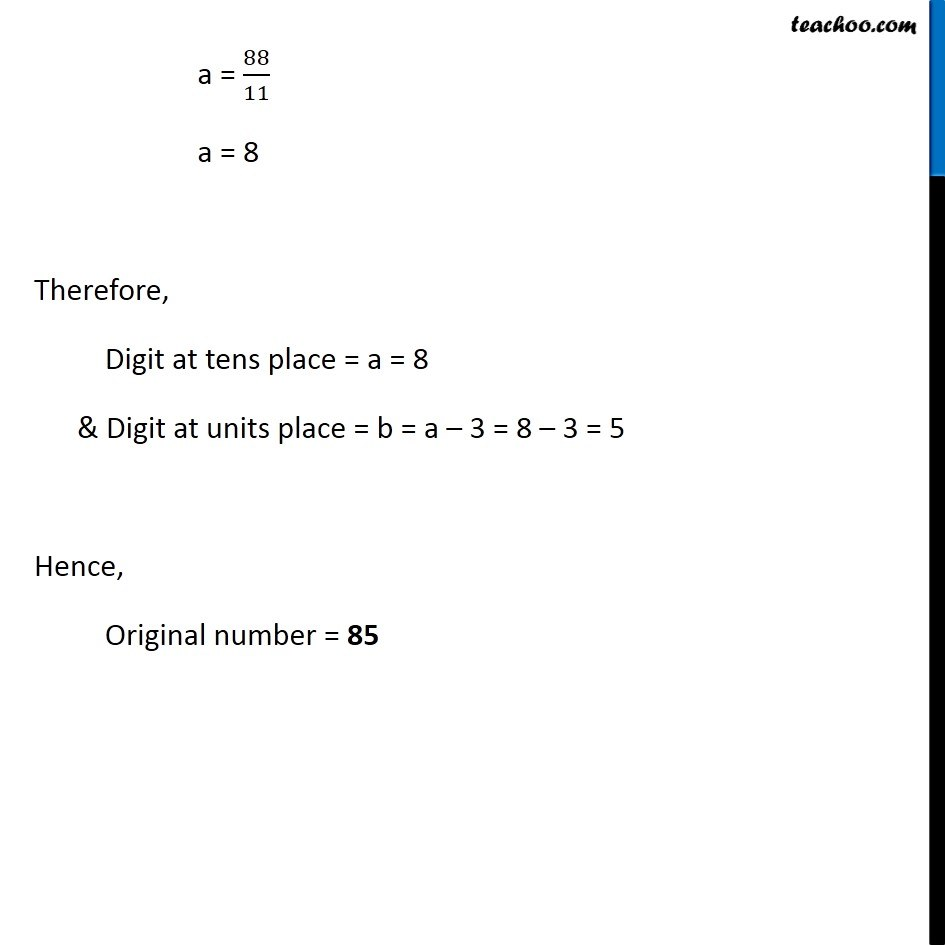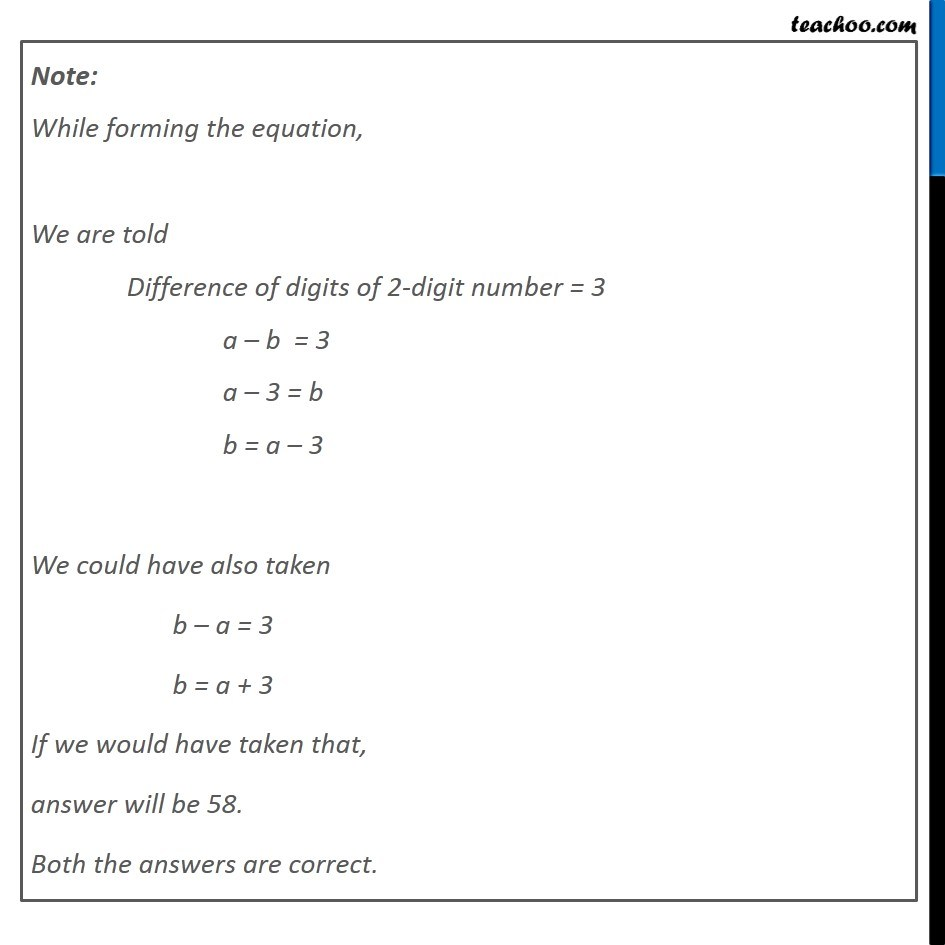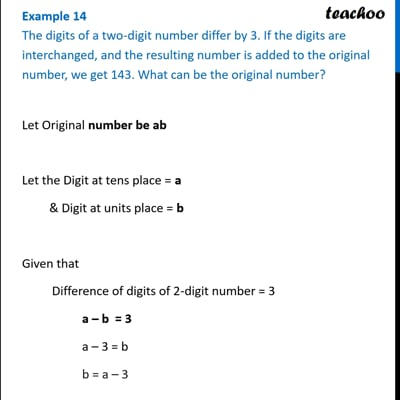This video is only available for Teachoo black users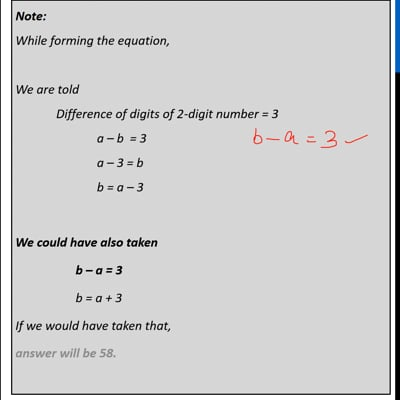This video is only available for Teachoo black users

Learn in your speed, with individual attention - Teachoo Maths 1-on-1 Class

### Transcript

Question 12 The digits of a two-digit number differ by 3. If the digits are interchanged, and the resulting number is added to the original number, we get 143. What can be the original number?Let original number be ab Let the digit at tens place = a & digit at units place = b Given that Difference of digits of 2-digit number = 3 a b = 3 a 3 = b b = a 3 Original Number ab Digit at units place = b = a 3 Digit at tens place = a Original number = (10 Digit at tens place) + (1 Digit at units place) = (10 a) + (1 (a 3)) = 10a + a 3 = 11a 3 Reverse number ba Digit at units place = a Digit at tens place = b = a 3 Reverse number = (10 Digit at tens place) + (1 Digit at units place) = 10 (a 3) + (1 a) = 10a 30 + a = 11a 30 Hence, Original number = 11a 3 Reverse number = 11a 30 Given that, When reverse number is added to original number , we get 143 Reverse number + Original number = 143 (11a 3) + (11a 30) = 143 11a + 11a 3 30 = 143 22a 3 30 = 143 22a = 143 + 3 + 30 22a = 166 a = 166/22 a = 88/11 a = 8 Therefore, Digit at tens place = a = 8 & Digit at units place = b = a 3 = 8 3 = 5 Hence, Original number = 85 Note: While forming the equation, We are told Difference of digits of 2-digit number = 3 a b = 3 a 3 = b b = a 3 We could have also taken b a = 3 b = a + 3 If we would have taken that, answer will be 58. Both the answers are correct.